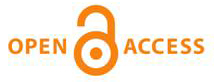Founder: Northern (Arctic) Federal University named after M.V. Lomonosov

Editorial office address: Russian Federation, 163002, Arkhangelsk, Naberezhnaya Severnoy Dviny 17, office 1410a

Phone: (818-2) 21-61-00(15-33)
e-mail: l.zhgileva@narfu.ru
http://aer.narfu.ru/en/

16+

# Maximal Prefix Codes and Subclasses of the Context-Free Languages Class. P. 121–129Section: Physics. Mathematics. Informatics

519.713

### Authors

Korabel’shchikova Svetlana Yur’evna
Institute of Mathematics, Information and Space Technologies, Northern (Arctic) Federal University named after M.V. Lomonosov (Arkhangelsk, Russia)
e-mail: kmv@atnet.ru
Mel’nikov Boris Feliksovich
Togliatti Branch of Samara State University (Togliatti, Russia)
e-mail: bormel@rambler.ru

### Abstract

This paper analyzes the relation between maximal prefix codes, formal language theory and alphabetic coding. Commutation conditions in the global supermonoid of the free monoid, equivalence criterion of a pair of finite languages and a range of other results, connected with infinite language iterations, are expressed in terms of maximal prefix codes. Many of these results are related to the algorithmic problems of monomial algebra (i.e. associative algebras defined by the so-called languages of obstructions). Prefix codes are mostly used in alphabetic coding, as the prefix property guarantees unambiguous decodability. Maximal prefix codes have a range of other properties: the MacMillan’s inequality becomes equality for them and all the knots of a code tree are saturated. We used the relation between the maximal prefix codes and the code trees and calculated the number of maximal prefix codes of the given power r in the q-alphabetic. In this paper we deduce the general formula and give its typical applications. The maximal prefix codes of power r over the q-alphabetic do not exist if the remainder on dividing r by q-1 isn’t equal to 1. The quotient k of r and q-1 can be explanated as the maximal quantity of tiers in the code tree and as the quantity of beams from q edges of the tree. The setup (n1, n2, n3, …, ns) represents the distribution of these beams over the tiers of the code tree. A number of unsolved problems and our conjectures of necessary conditions of commutation, requiring further verification, are given in the conclusion.

### Keywords

context-free language, prefix code, code tree, maximal prefix code

### References

1. Lallement G. Semigroups and Combinatorial Applications. Wiley, 1979. 376 p.
2. Mel’nikov B. The Equality Condition for Infinite Catenations of Two Sets of Finite Words. Int. J. Found. Comput. Sci., 1993, vol. 4, no. 3, pp. 267–274.
3. Mel’nikov B. Some Equivalence Problems for Free Monoids and for Subclasses of the CF-grammars Class. Number Theor. Algebr. Methods Comput. Sci., 1995, pp. 125–137.
4. Mel’nikov B.F. Opisanie spetsial’nykh podmonoidov global’nogo nadmonoida svobodnogo monoida [Special Submonoids Description of Global Supermonoid of Free Monoid]. Izv. vussh. ucheb. zavedeniy. Matematika, 2004, no. 3, pp. 46–56.
5. Mel’nikov B.F. Nekotorye sledstviya usloviya ekvivalentnosti odnoznachnykh skobochnykh grammatik [Some Consequences of Equivalence Condition of Unambiguous Parenthesis Grammar]. Vestn. Mosk. univ., Ser. 15, 1991, no. 3, pp. 51–53.
6. Dubasova O.A., Mel’nikov B.F. Ob odnom rasshirenii klassa kontekstno-svobodnykh yazykov [On an Extension of the Context-Free Languages Class]. Programmirovanie, 1995, no. 6, pp. 46–56.
7. Mel’nikov B., Kashlakova E. Some Grammatical Structures of Programming Languages as Simple Bracketed Languages. Informatica, 2000, vol. 11, no. 4, pp. 441–446.
8. Ginzburg S. Matematicheskaya teoriya kontekstno-svobodnykh yazykov [Mathematical Theory of the Context- Free Languages]. Moscow, 1970, 232 p.
9. Staneviciené L. Ob odnom sredstve issledovaniya beskontekstnykh yazykov [On a Research Instrument of Context-Free Languages]. Kibernetika, 1989, no. 4, pp. 135–136.
10. Staneviciené L. D-graphs in Сontext-Free Language Theory. Informatica Lithuanian Acad. Sci., 1997, vol. 8, no. 1, pp. 43–56.
11. Meytus V. Razreshimost’ problemy ekvivalentnosti determinirovannykh magazinnykh avtomatov [Equivalence Problem Solubility of Deterministic Pushdown Automaton]. Kibernetika i sistem. analiz, 1992, no. 5, pp. 20–45.
12. Sénizergues G. L(A)=L(B)? Decidability Results From Complete Formal Systems. Theor. Comput. Sci., 2001, vol. 251, no. 1–2, pp. 1–166.
13. Mel’nikov B.F. Podklassy klassa kontekstno-svobodnykh yazykov [Subclasses of a Context-Free Languages Class]. Moscow, 1995. 160 p.
14. Mel’nikov B. 2ω-Finite Automata and Sets of Obstructions of Their Languages. Korean J. Comput. Appl. Math., 1999, no. 6, pp. 23–28.
15. Mel’nikov B.F. Ob ω-yazykakh spetsial’nykh billiardov [On the Special Billiards ω-Languages ]. Diskret. matematika, 2002, no. 3, pp. 95–105.
16. Mel’nikov B. On an Expansion of Nondeterministic Finite Automata. J. Appl. Math. Comp., 2007, vol. 24, no. 1–2, pp. 155–165.
17. Dragovich V., Radnovich M. Integriruemye billiardy, kvadriki i mnogomernye porizmy Ponsele [Integrable Billiards, Quadrics and Multidimensional Poncelet Porisms]. Moscow, 2010. 338 p.
18. Ufnarovskiy V. Kombinatornye i asimptoticheskie metody v algebre [Combinatorial and Asymptotic Methods in Algebra]. Itogi Nauki Tekhn. Ser.: Sovr. probl. matematiki. Fundament. napravleniya, 1990, vol. 6, pp. 5–177.
19. Belov A., Borisenko V., Latyshev V. Monomial’nye algebry [Monomial Algebra]. Itogi Nauki Tekhn. Ser.: Sovr. mat. i ee pril. Tematicheskie obzory, 2002, vol. 26, pp. 35–214.
20. Korabel’shchikova S.Yu., Chesnokov A.I. Ob odnom algoritme opredeleniya kolichestva tsiklicheskikh kodov [On a Number of Cyclic Codes Algorithm]. Mezhdistsiplinarnye issled. v oblasti mat. modelirovaniya i informatiki: materialy 3-y nauch.-prakt. internet-konf. [Multidisciplinary Research in the Math. Model and Computer Sci.: Proc. of the 3rd Sci. and Practical Internet-Conf.]. Ulyanovsk, 2014. pp. 37–41.
21. Korabel’shchikova S.Yu., Chesnokov A.I. O chisle razlichnykh tsiklicheskikh kodov zadannoy dliny [On a Number of Different Cyclic Codes of the Specified Length]. Vektor nauki TGU, 2013, no. 4(26), pp. 25–26.
22. Zyablitseva L.V., Korabel’shchikova S.Yu., Chesnokov A.I. Lineynye kody, ispravlyayushchie oshibki, i algoritmy ikh podscheta [Linear Error-Correcting Codes and Algorithms for Their Calculations]. Evrist. algoritmy i raspredelennye vychisleniya, 2014, vol.1, no. 3, pp. 47–59.
23. Yablonskiy S.V. Vvedenie v diskretnuyu matematiku: ucheb. posobie dlya studentov vuzov [Introduction to Discrete Mathematics]. Moscow, 2002. 384 p.
24. Gavrilov G.P., Sapozhenko A.A. Zadachi i uprazhneniya po diskretnoy matematike [Exercises on Discrete Mathematics]. Moscow, 2004. 416 p.